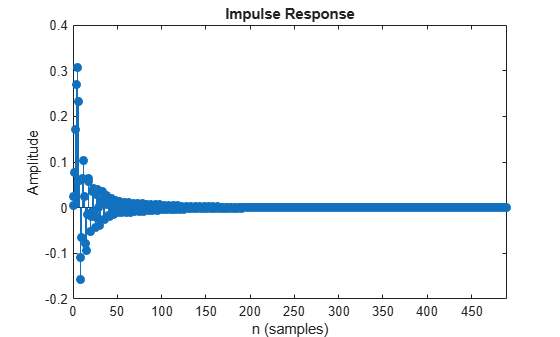impz

Impulse response of discrete-time filter System object

Description

example

[impResp,t] = impz(sysobj) computes the impulse response of the filter System object™, sysobj, and returns the response in column vector impResp, and a vector of times (or sample intervals) in t, where t = [0 1 2 ...k-1]'. k is the number of filter coefficients.

[impResp,t] = impz(sysobj,n) computes the impulse response at floor(n) one-second intervals. The time vector t equals (0:floor(n)-1)'.

[impResp,t] = impz(sysobj,n,fs) computes the impulse response at floor(n) 1/fs-second intervals. The time vector t equals (0:floor(n)-1)'/fs.

[impResp,t] = impz(sysobj,[],fs) computes the impulse response at k 1/fs-second intervals. k is the number of filter coefficients. The time vector t equals (0:k-1)'/fs.

[impResp,t] = impz(sysobj,'Arithmetic',arithType) computes the impulse response based on the arithmetic specified in arithType, using either of the previous syntaxes.

impz(sysobj) uses fvtool to plot the impulse response of the filter System object sysobj.

You can use impz for both real and complex filters. When you omit the output arguments, impz plots only the real part of the impulse response.

For more input options, refer to impz in Signal Processing Toolbox™.

Examples

collapse all

Create a discrete-time filter for a fourth-order, lowpass elliptic filter with a cutoff frequency of 0.4 times the Nyquist frequency. Use a second-order sections structure to resist quantization errors. Plot the first 50 samples of the impulse response, along with the reference impulse response.

d = fdesign.lowpass(.4,.5,1,80);

Create a design object for the prototype filter. Use ellip to design a minimum order discrete-time biquad filter.

Plot the impulse response.

axis([1 75 -0.2 0.35])Input Arguments

collapse all

Length of the impulse response vector, specified as a positive integer.

Data Types: single | double | int8 | int16 | int32 | int64 | uint8 | uint16 | uint32 | uint64

Sampling frequency used in computing the impulse response, specified as a positive scalar.

Data Types: single | double | int8 | int16 | int32 | int64 | uint8 | uint16 | uint32 | uint64

Arithmetic used in the filter analysis, specified as 'double', 'single', or 'Fixed'. When the arithmetic input is not specified and the filter System object is unlocked, the analysis tool assumes a double-precision filter. When the arithmetic input is not specified and the System object is locked, the function performs the analysis based on the data type of the locked input.

The 'Fixed' value applies to filter System objects with fixed-point properties only.

When the 'Arithmetic' input argument is specified as 'Fixed' and the filter object has the data type of the coefficients set to 'Same word length as input', the arithmetic analysis depends on whether the System object is unlocked or locked.

• unlocked –– The analysis object function cannot determine the coefficients data type. The function assumes that the coefficients data type is signed, has a 16-bit word length, and is auto scaled. The function performs fixed-point analysis based on this assumption.

• locked –– When the input data type is 'double' or 'single', the analysis object function cannot determine the coefficients data type. The function assumes that the data type of the coefficients is signed, has a 16-bit word length, and is auto scaled. The function performs fixed-point analysis based on this assumption.

To check if the System object is locked or unlocked, use the isLocked function.

When the arithmetic input is specified as 'Fixed' and the filter object has the data type of the coefficients set to a custom numeric type, the object function performs fixed-point analysis based on the custom numeric data type.

Output Arguments

collapse all

Impulse response, returned as an n-element vector. If n is not specified, the length of the impulse response vector equals the number of coefficients in the filter.

Data Types: double

Time vector of length n, in seconds. t consists of n equally spaced points in the range (0:floor(n)-1)'/fs. If n is not specified, the function uses the number of coefficients of the filter.

Data Types: double